# VECM + Neural Network: A semiparametric model of cointegrated data

In this article, I explore a method of nonlinear time series estimation, which combines elements of an artifical neural network (NN) and a vector error correction model (VECM). The aim is to develop a semiparametric VECM, which is capable of modelling nonlinear short-run behaviour of an unknown functional form, while retaining the ability to draw inferential conclusions about the long-run equilibrium behaviour of the data. This approach is particularly useful for data periods including financial crises, multiple regimes and other nonlinear characteristics, which are difficult to handle in a purely linear setting.

The artificial neural network (NN) is a powerful tool for modeling nonlinear empirical relationships. Various authors demonstrate the so-called Universal Approximation Theorem (see for instance Hornik (1991)), proving that single-layer neural networks can approximate any arbitrary function. This makes it an elegant alternative to other nonlinear approaches — particularly, when the functional form of the data-generating process (DGP) is unknown.

Using artificial neural networks to capture nonlinearities in time series data has received a fair amount of attention in the past. Autoregressive neural network (AR-NN) models are well established and have been applied broadly (see Enders (2015)). Various studies compare the performance of multivariate neural network (VAR-NN) models against standard vector autoregression models (see Wutsqa, Subanar, and Sujuti (2006) and Aydin and Cavdar (2015)). Generally, NN-based models exhibit superior performance for prediction purposes, while this comes at the expense of model inference, given the “black-box” nature of the NN component. As always, there is no free lunch in econometrics.

## Introducing the VEC-NN

Very little prior work has been done to apply neural networks to cointegrated data in VEC-NN models, and thus the purpose of this article is exploratory: I assess a battery of different specifications with the aim of exploring the merits of each. My ultimate goal is to develop a flexible semiparametric formulation of the VECM, which permits some inference about the long-run.

In the following section, I examine 7 distinct models, with varying degree of nonlinear behaviour.

### Model 1: Basic VECM

The basic VECM model takes the following form:

$\begin{equation} \Delta Y_t = \mu_0 + \Pi Y_{t-1} + \sum_{i=1}^{p} \Gamma_{i} \Delta Y_{t-i} + \varepsilon_t \tag{1} \end{equation}$

Where $$\Pi$$ can be decomposed into $$\alpha \beta'$$, when the series are cointegrated. In NN syntax, the VECM can be described as a set of input nodes linked directly to output nodes via weights and no hidden layer. Figure 1 depicts the VECM using a signal-flow diagram, which is useful in visualizing the approach: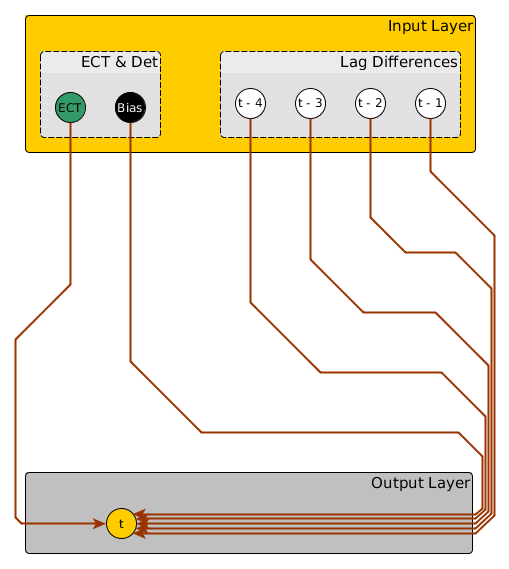Figure 1: VECM signal-flow diagram

This model represents the linear base case against which all further enhancements are benchmarked.

### Model 2: VEC-NN (nonlinear $$\Gamma$$-matrix)

Introducing a single-layer neural network with $$H$$ hidden nodes and an activation function $$\Psi(\cdot)$$ to Eq. (1), in order to capture the short-run nonlinear behaviour, allows us to re-specify the model as follows:

$\begin{equation} \Delta Y_t = \Pi Y_{t-1} + \sum_{j=1}^{H} \Psi (m_{j0} + \sum_{i=1}^{p} B_{ij} \Delta Y_{t-i}) \gamma_j + \varepsilon_t \tag{2} \end{equation}$

This model, like the standard VECM, can be estimated in a maximum likelihood framework, and is represented by a signal-flow diagram in Figure 2. The diagram illustrates the point made above: that the VECM can be interpreted as a variant of a NN where all input nodes skip the hidden layer entirely. In Figure 2 some of the connections are now ‘re-routed’ through the hidden layer. As we will see, different specifications simply allow different elements (Lag differences and/or ECT) to feed into the hidden layer.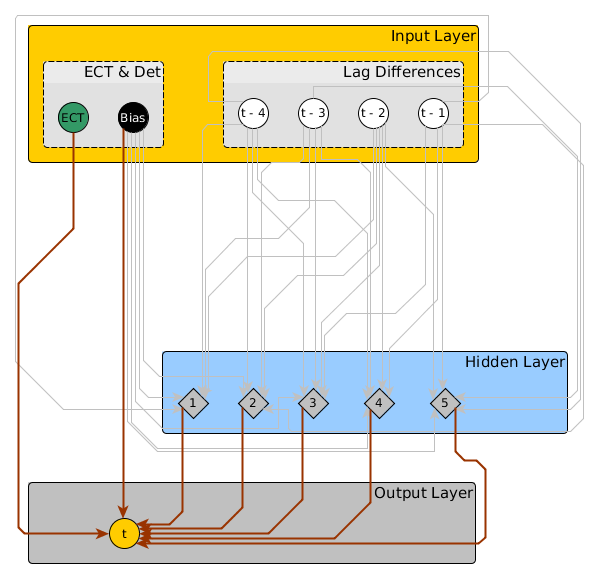Figure 2: VEC-NN signal-flow diagram

### Model 3: VEC-NN (linear and nonlinear $$\Gamma$$-matrix)

A further variant of this modelling approach builds on the logic applied by Lee, White, and Granger (1993) and others, who estimate a linear specification augmented by a neural network capturing any remaining nonlinearities. This ‘augmented’ model has the advantage of providing an attractive modelling philosophy by starting with a linear model and incrementally adding nonlinear elements as justified by the data (particularly when combined with an empirical test for remaining nonlinearity):

$\begin{equation} \Delta Y_t = \mu_0 + \Pi Y_{t-1} + \sum_{i=1}^{p} \Gamma_{i} \Delta Y_{t-i} + \sum_{j=1}^{H} \Psi (m_{j0} + \sum_{i=1}^{p} B_{ij} \Delta Y_{t-i}) \gamma_j + \varepsilon_t \tag{3} \end{equation}$

Both of the above approaches (Eq. (2) & (3)) have the compelling benefit of retaining the $$\Pi$$-matrix, which may allow us to perform cointegration tests and/or to extract $$\alpha$$- and $$\beta$$-matrices.

### Model 4 & 5: VEC-NN (nonlinear $$\alpha$$-vector)

Another modelling approach is introduced by Dietz (2010), which allows for nonlinear interactions between the short- and long-run components of the model. The specification takes the following form:

$\begin{equation} \Delta Y_t = \mu_0 + \sum_{j=1}^{H} \Psi (\beta' Y_{t-1})\gamma_j + \sum_{i=1}^{p} \Gamma_{i} \Delta Y_{t-i} + \varepsilon_t \tag{4} \end{equation}$

Again, this can be modelled in augmented form:

$\begin{equation} \Delta Y_t = \mu_0 + \Pi Y_{t-1} + \sum_{j=1}^{H} \Psi (\beta' Y_{t-1})\gamma_j + \sum_{i=1}^{p} \Gamma_{i} \Delta Y_{t-i} + \varepsilon_t \tag{5} \end{equation}$

The drawback of the above approach is that a priori knowledge of the cointegrating rank and/or vectors is necessary. Dietz (2010) suggests imposing a structural cointegrating vector estimated by 3SLS, but this remains somewhat unsatisfactory. I introduce a method which estimates the cointegrating vector, but requires prior knowledge of the rank of the $$\Pi$$-matrix. A practical issue in the implementation is that estimation requires the optimization of a non-differentiable likelihood function, which is computationally slow.

### Model 6 & 7: VEC-NN (nonlinear $$\alpha$$ and $$\Gamma$$)

Finally, the models can be combined into a specification where all short-run parameters, including $$\alpha$$ are modelled by a nonlinear process. Again, this can be augmented by the linear model. Drawbacks and estimation issues are similar to models 4 & 5, and the equations are omitted here, since they simply represent combinations of the models outlined above.

## Putting the method to the test

### Estimation

The practical implementation of the method makes use of the ‘nnet’-package in R, which can be configured to estimate the VEC-NN on an equation-by-equation basis1. Rank restrictions, which are necessary to model the short- and long-run interactions ($$\alpha$$) are imposed in a joint likelihood function which is solved using the SANN optimizer2. The restricted estimation is computationally expensive, and the SANN optimizer is slow and somewhat inefficient. The approach is sufficient for illustrative purposes, but more work needs to be done to develop a better solution.

### Lag Selection & Overfitting

For demonstration purposes, I simply impose the correct (simulated) lag-order in this analysis (4 lags), however lag-selection remains possible in the VEC-NN setting using a fitting criterion such as the network information criterion (NIC). The NIC is a generalization of the AIC, which is suitable for use with neural networks.

To prevent overfitting, it is possible to limit the number of hidden nodes and to set a weight decay factor, both of which are determined using a cross validation. Since the model is currently estimated on an equation-by-equation basis, the fitting criterion is summed across all equations with a single value for $$H$$ and the decay factor imposed on the entire system, rather than allowing an equation-specific cross-validation. Finally, a linear activation function is used to model connection between the hidden and output layers. Bounded activation functions, such as the sigmoid or tanh function, can also be used with appropriate scaling, however this is left for future exploration.

### Data Simulation

To test the specifications, I simulate a bivariate cointegrated system of equations with multiple thresholds in the short-run (Threshold VECM) and 4 lags. There exists a fixed cointegrating relationship of $$\beta = [1,-1]$$, and the thresholds introduce some degree of nonlinearity to the system. Figure 3 displays the series graphically: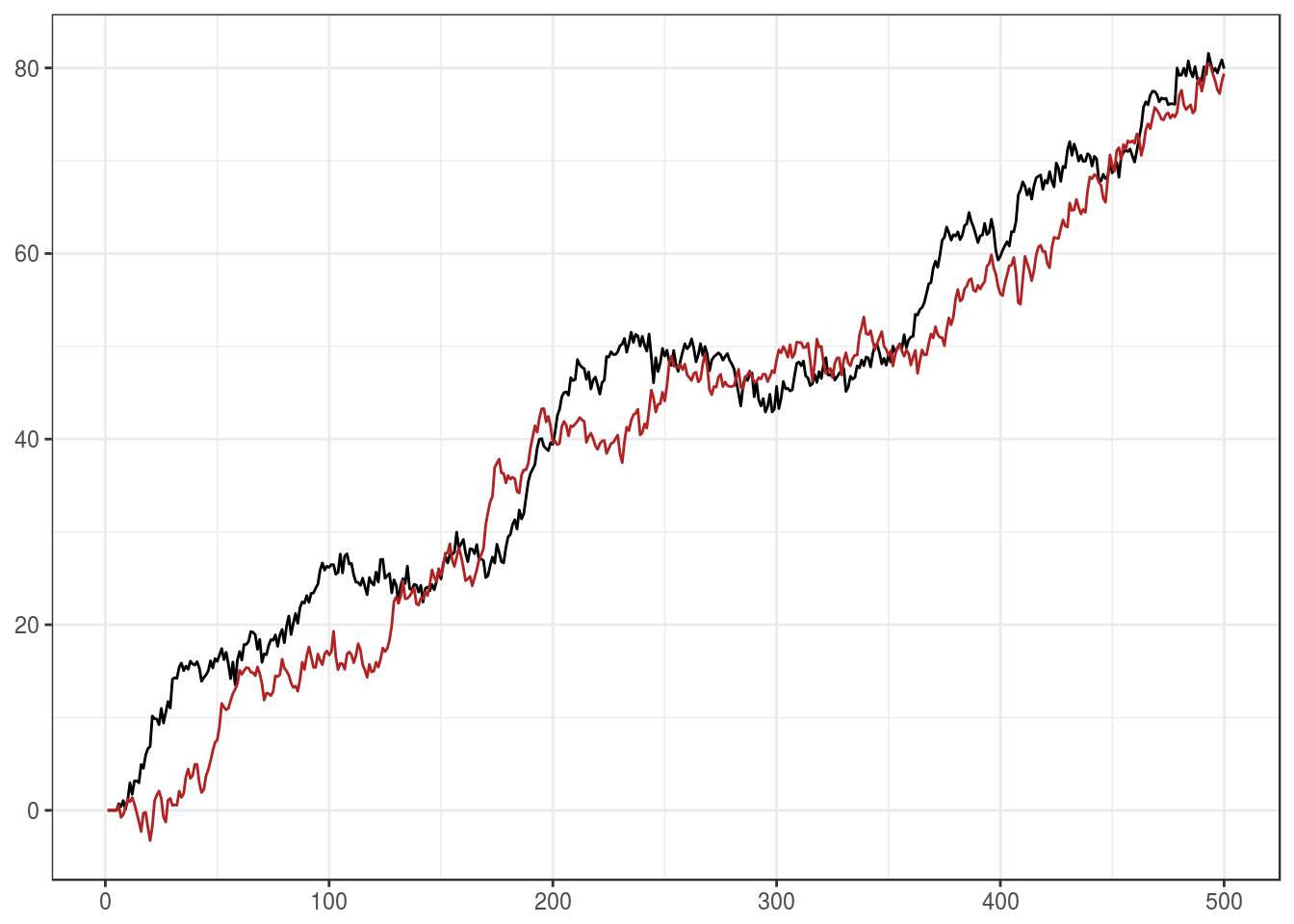Figure 3: Simulated cointegrated series

In order to assess, how well the linear and nonlinear specifications perform in capturing the simulated dynamics, the data is split into a training and test set, with the initial 90% of periods in the training sample.

### Statistical Fit

The first step, is to assess the out-of-sample fit of the various models. I use the average RMSE3 as a statistical measure of fit, and plot the results of Models 1-7, as well as a VAR model, which ignores the long-run ECT, in Figure 4: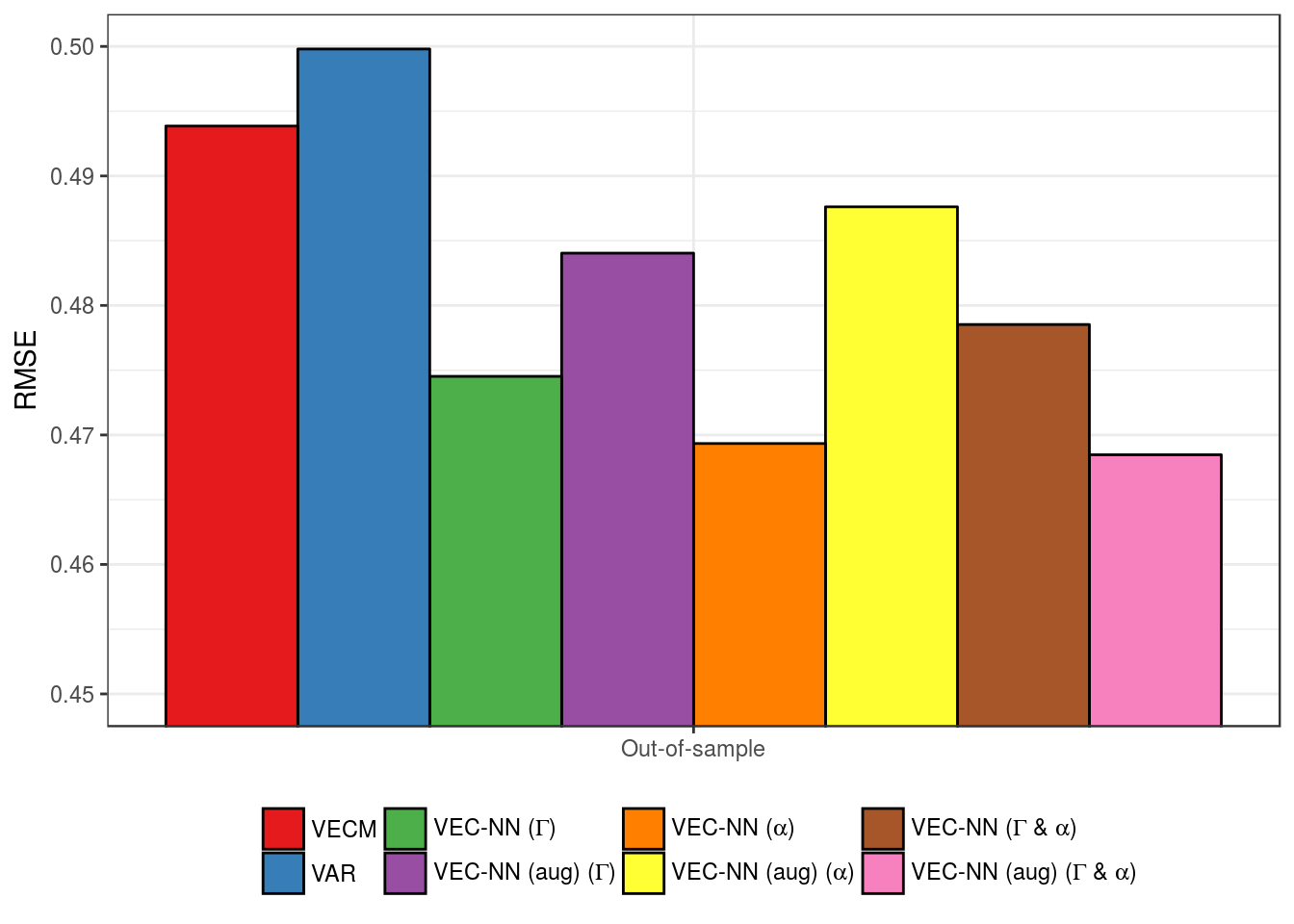Figure 4: Estimation Fit (Out-of-sample RMSE)

A few general observations can be made from the above result. Firstly, all models outperform the linear VECM. This is encouraging, and demonstrates that the NN does indeed provide a feasible framework to capture the nonlinearities in the data. As may be expected, the VAR represents a worse fit than the VECM, given the cointegrated nature of the DGP.

A second noteworthy observation is that the fit of the linear augmented models is worse for 2 of 3 variations. This is not surprising, since the DGP consists of 3 separate linear processes and not of a single linear process overlayed with nonlinear features. As such, while the linear augmented VEC-NN is an elegant approach, and does provide superior outcomes, the choice of model needs to be informed by the postulated structure of the DGP.

Finally, there does not seem to exist a fundamental difference between the nonlinear variants. The cross-validation applied in the estimation searches over a fairly narrow grid of tuning parameters, favouring speed over precision, and thus potentially obscuring nuances by sub-optimal size and decay selection. Nonetheless, the models all appear to be suitable to capture the threshold behaviour in the simulated dataset.

Since this result is based on one simulated dataset only, I perform a robustness check by simulating 100 datasets and measuring the out-of-sample RMSE for models 1 (VECM), 2 (VEC-NN ($$\Gamma$$)) and 3 (VEC-NN (aug) ($$\Gamma$$)). $$\alpha$$-vector variants are omitted due to the computational cost of running a Monte Carlo analysis. Figure 5 displays the outcome: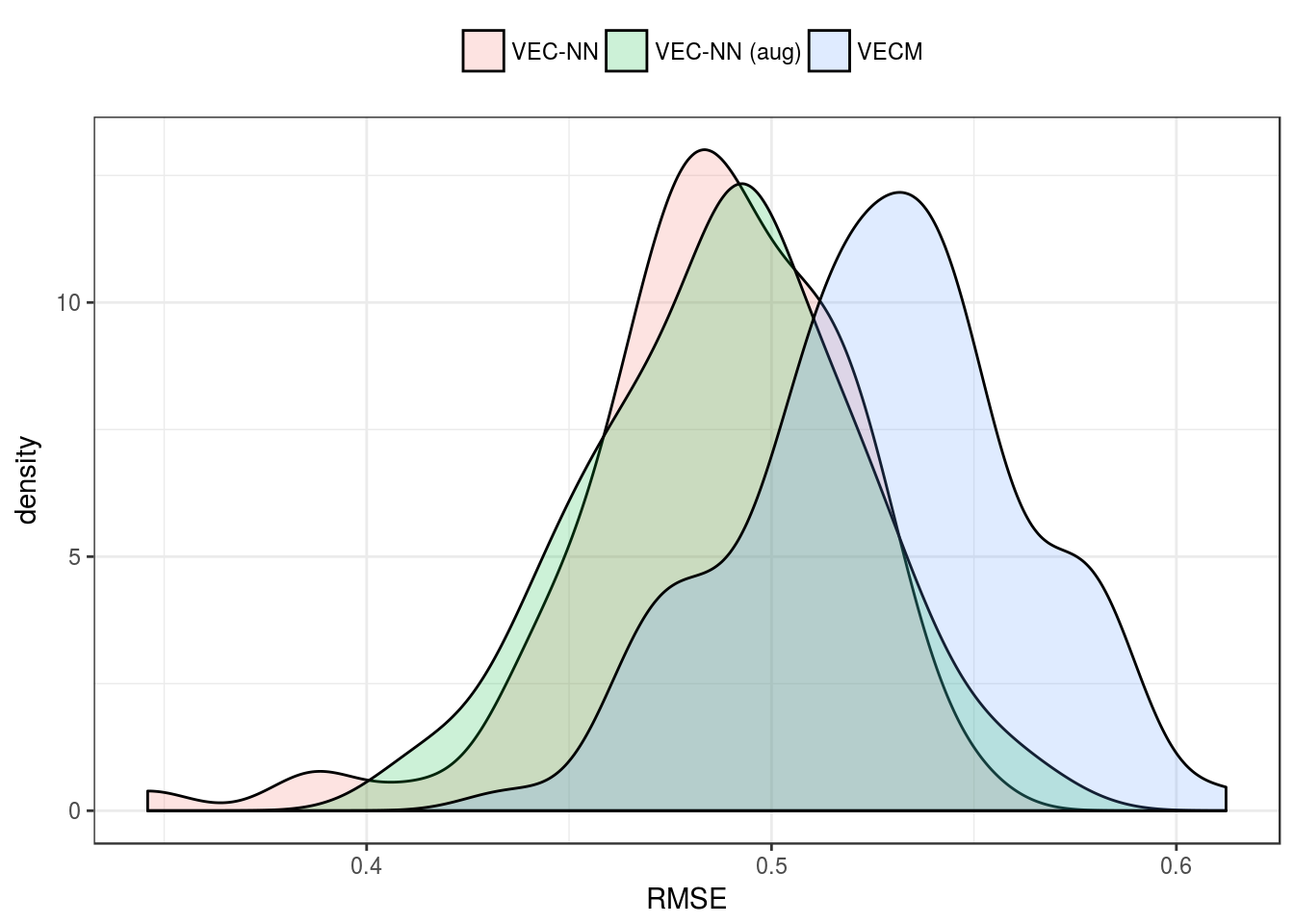Figure 5: Monte Carlo simulation of model fit

The distribution of the RMSE is distinctly lower for both nonlinear models, indicating a high degree of robustness in the above findings.

### Long-run Inference

A test for cointegration may be possible by examining the rank of the $$\Pi$$-matrix, which is produced by models 2 & 3. Potential approaches include information criteria tests, cross validation, or a version of the Johansen procedure (Camba-Mendez and Kapetanios 2008; Dietz 2010). I postpone further discussion to later articles however, and limit myself to exploring the framework more broadly in the current context.

Instead of testing for cointegration, I impose a rank restriction of $$r = 1$$ on the $$\Pi$$-matrix. This is a cross-equation restriction that is implemented by maximising a joint likelihood function for the system. Due to the computational cost, only model 2 & 4 are evaluated below (no linear augmented models). I choose arbitrary tuning parameter values ($$H = 10$$, $$decay = 0.1$$), again, to avoid excessive processing time. Recall, that the true $$\beta$$-vector is equal to $$[1,-1]$$. The models are estimated by imposing a restriction on the first parameter, and estimating the second ($$-1$$). Figure 6 presents the outcome of the Monte Carlo experiment: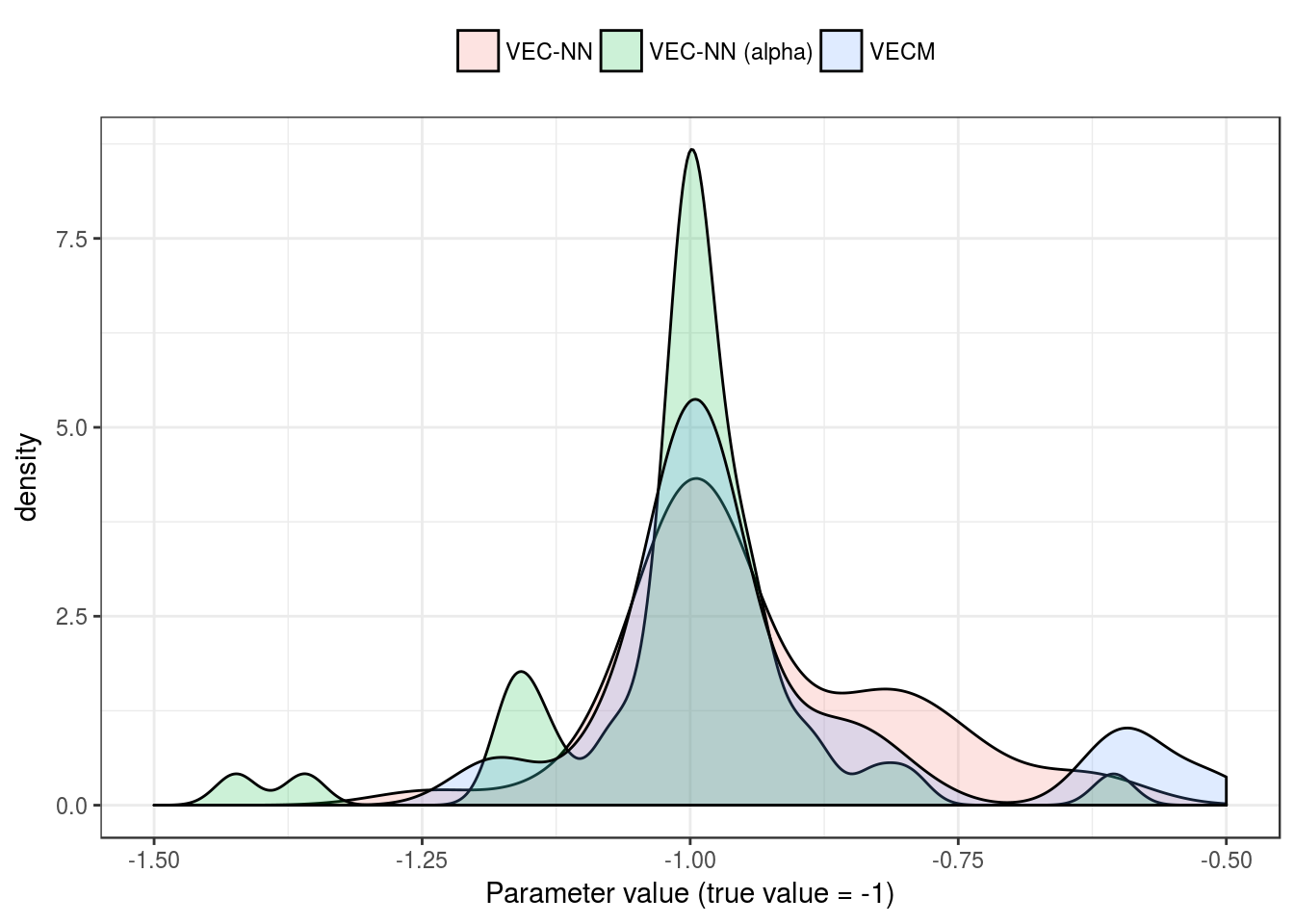Figure 6: ECT parameter estimation

The results show that the nonlinear models consistently obtain the correct parameter value. There is a degree of variability in the estimate for all specifications, but the nonlinear models generally perform similarly to the linear model. This is despite the arbitrary selection of tuning parameters of the nonlinear models. Model 4 obtains the correct value most frequently, indicating the usefulness of modelling the interaction between the short- and long-run parameters. Model 2 also displays consistent values, with the added benefit of cointegration testing in this framework. However, a cross-validation or information criteria based method of cointegration testing (mentioned above) may apply to both, model 2 and 4 equally.

## Next steps

This article has provided a brief introduction to a method of flexibly estimating combinations of neural networks and vector error correction models. Various extensions and performance issues remain to be explored, and will be addressed in further work. When using a sigmoid or tanh activation function, the VEC-NN resembles a type of multivariate smooth-transition threshold model with regime switching mean. A comparison between these methods may be a worthwhile addition.

As mentioned, a systematic ‘Box-Jenkins’ type modeling approach is possible, and warrants more detailed discussion. Particularly, issues of lag selection and model evaluation are largely unexplored here. Cointegration testing may be interesting in a modified version of the Johansen procedure which treats the VEC-NN as an essentially parameterised model.

Various possibilities remain, to explore patterns in the short-run of the model. Running parameter sensitivity tests can provide a notion of the directional response of the model to shocks to a variable at different levels of the remaining variables. Variable importance measures can provide some further insights into the dynamics of the model, particularly as potential nonlinear alternatives to Granger-causality tests.

The above points largely represent blue-sky thinking, and I have not completed any detailed analysis on them. Nonetheless, they provide an inkling of the direction and types of topics that can be discussed in the context of VEC-NN. In general, the method appears to be a promising candidate to answer business-cycle related questions, as well as various other topics which exhibit asymmetric or regime switching behaviour.

1. Estimating the model in a multivariate setting is also possible and will be implemented soon. The benefit of multivariate estimation lies in potentially shorter calculation time when imposing cross-equation restrictions.

2. BFGS does not perform well — likely due to the reliance on differentiability.

3. The scale of the residuals is normalised, in order to make the errors comparable across series.